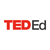# What happens if you guess? - Leigh Nataro

• 606,554 Views
• TEDEd Animation

#### Let’s Begin…

Will it rain tomorrow? How likely is your favorite team to win the Super Bowl? Questions like these are answered through the mathematics of probability. Watch this artistic visualization of your odds of passing a test if you don’t know any of the answers.

178

Create and share a new lesson based on this one.

TED-Ed Animations feature the words and ideas of educators brought to life by professional animators. Are you an educator or animator interested in creating a TED-Ed Animation? Nominate yourself here »

## Meet The Creators

• Educator Leigh Nataro
• Animator Matthew Saunders
• Narrator Leigh Nataro
Additional Resources for you to Explore
1) This lesson focused on the answer to a question being either right (success) or wrong (failure). When a result can fall into one of two categories (success or failure), there are a fixed number of independent trials (for example, exactly 10 questions) and the probability of success is the same from trial to trial (for example, ½), this is considered a binomial situation. Which of these situations is binomial? Explain. Situation 1: A fair six-sided die is tossed three times. What is the probability of having all the rolls show a “4”. Situation 2: A fair-six sided die is tossed until a “4” appears. What is the probability of it taking 7 or more tosses?
2) On a True-False quiz there are two options – true or false. That means the probability of getting all questions right by randomly guessing is the same as the probability of getting all questions wrong by randomly guessing. Now think about a multiple choice question with three options – A, B or C. Do you think the probability of getting all questions right by randomly guessing is the same as the probability of getting all questions wrong by randomly guessing? Explain.
Links to websites involving binomial probability:
http://stattrek.com/online-calculator/binomial.aspx
http://www.rossmanchance.com/iscam2/applets/BinomDist/BinomDist.htmlTED-Ed
Lesson Creator
New York, NY

Create and share a new lesson based on this one.